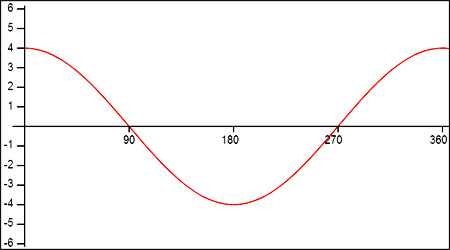Graphs of Sinusoids to Equations
View the Lesson | MATHguide homepage Updated January 15th, 2017

Status: Waiting for your answers.Determine the characteristics of the sinusoid above and fill in the information below using degrees. Enter the equation of the sinusoid in the form:
y = a sin bx     or     y = a cos bx.

 Amplitude : Period : Interval : [, ] y-intercept : (, ) Equation : y = pick sin cos x